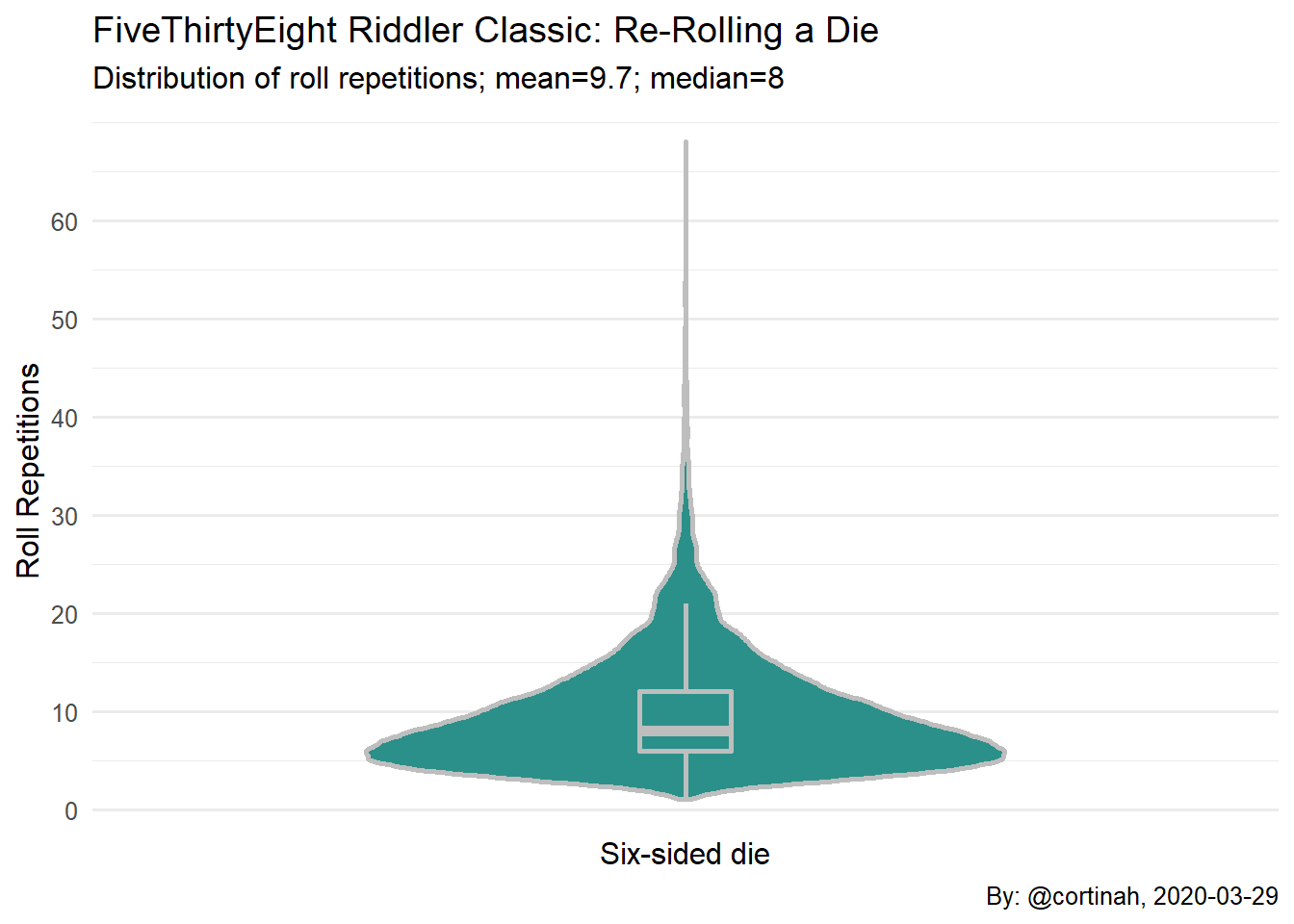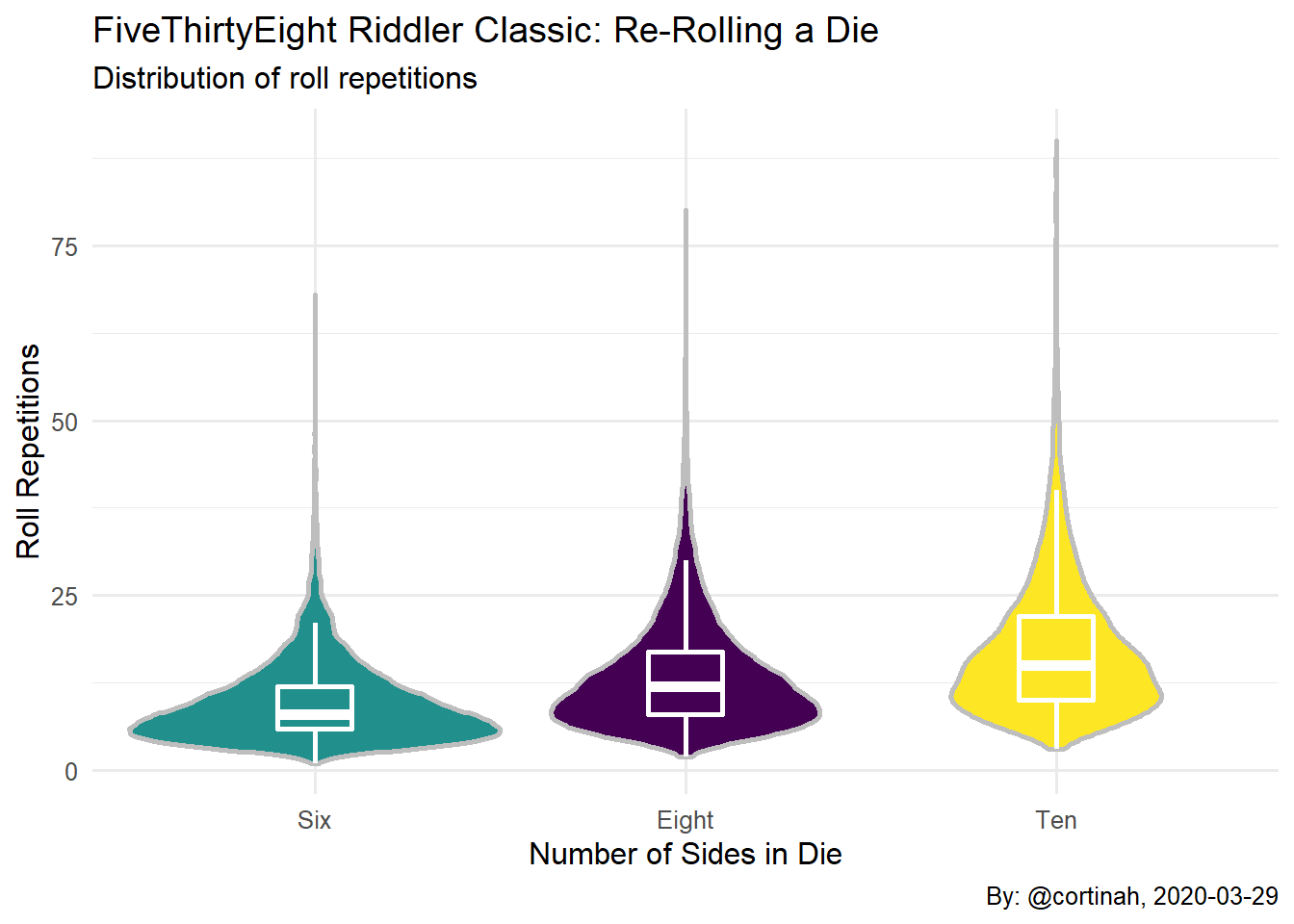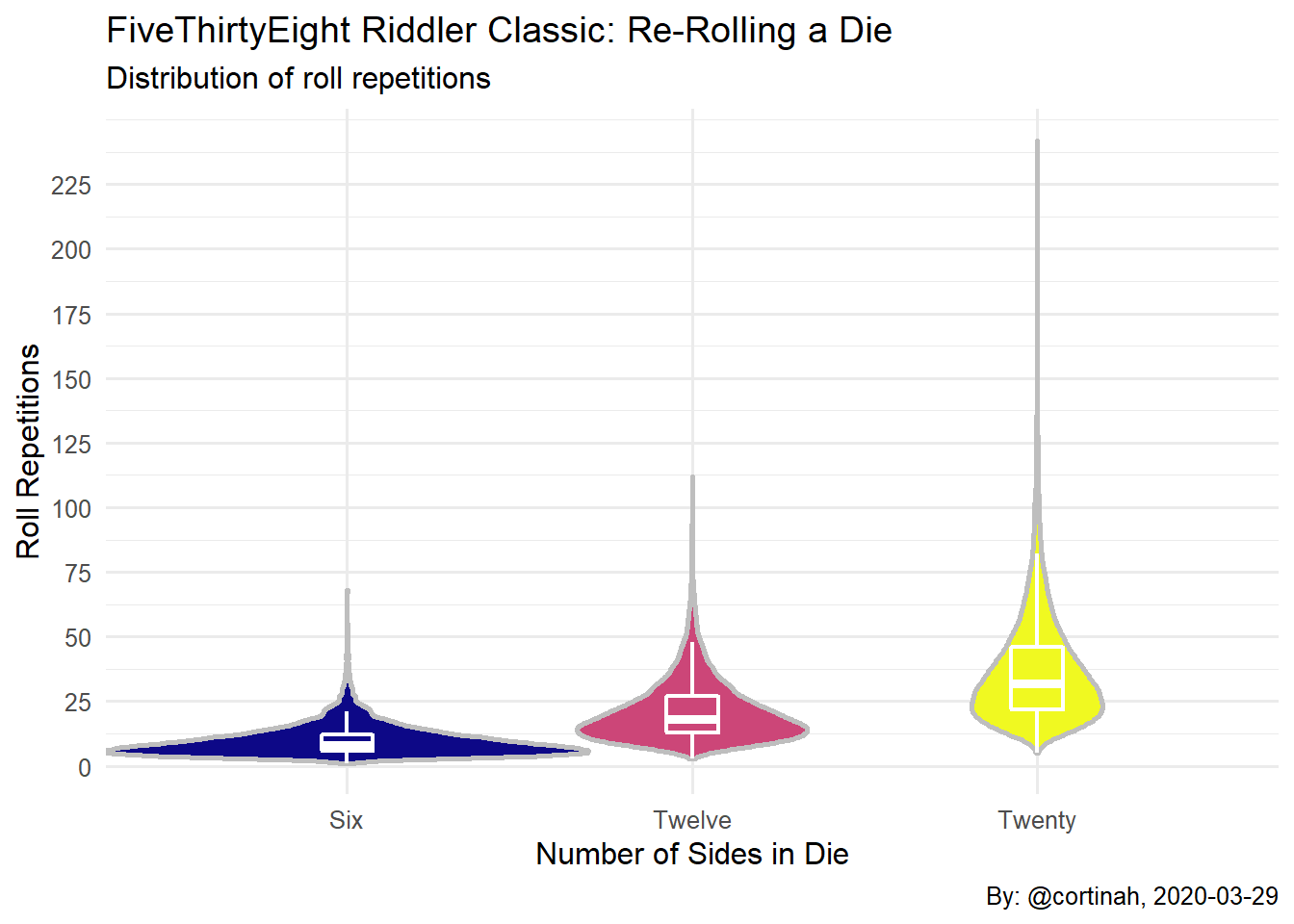# Riddler Classic: Re-Rolling a Die

## Rolling (and Re-Rolling) a Die:

In this week’s FiveThirtyEight Riddler Classic we start with a fair six-sided die and roll it six times, recording the results of each roll. We write these numbers on the six faces of another fair die. Next, we roll this second die six times and take those six numbers and write them on the faces of yet another die, continuing this process of generating a new die from the previous one. The question is: what is the average number of rolls it will take to get a die with the same number on all its faces?

## Proposed Solution:

While an analytical solution seems feasible, simulating and plotting this process is quite straightforward. Simulating also makes it simple to generalize to n-sided dies. The R code below loops through the six die rolls, assigning the results to the faces of a new die, until all of the faces have the same number. We look at combined violin/boxplots of the simulation.

``````dice_game <- function(n=6) {
rolls <- sample(n, n, replace=TRUE)
unique_rolls <- unique(rolls)
count <- 1

while(length(unique_rolls) > 1) {
rolls <- sample(rolls, n, replace=TRUE)
unique_rolls <- unique(rolls)
count <- count + 1
}
return(count)
}``````

We play the dice game 20,000 times and find that the average number of times the dice are rolled is 9.7 with a standard error of 0.04. The median number of times the dice are rolled is 8.

``````set.seed(12345)
games <- replicate(20000, dice_game(), simplify = T)
mean(games)``````
``##  9.71325``
``fivenum(games)``
``##   1  6  8 12 68``
``sd(games)/sqrt(length(games))``
``##  0.0420899``

## Violin Plot of the Six-Sided Simulation:

As shown, most games last between six and twelve rounds, but particularly skilled dice throwers can have games that last nearly seventy rounds, or even just one round.